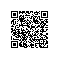# 数字精确运算BigDecimal常用方法

import java.math.BigDecimal;

public class Arith {

/**
* 由于Java的简单类型不能够精确的对浮点数进行运算，这个工具类提供精
* 确的浮点数运算，包括加减乘除和四舍五入。
*/
//默认除法运算精度
private static final int DEF_DIV_SCALE = 10;

//这个类不能实例化
private Arith(){

/**
* 提供精确的加法运算。
* @param v1 被加数
* @param v2 加数
* @return 两个参数的和
*/
public static double add(double v1,double v2){
BigDecimal b1 = new BigDecimal(Double.toString(v1));
BigDecimal b2 = new BigDecimal(Double.toString(v2));
return b1.add(b2).doubleValue();
}
/**
* 提供精确的减法运算。
* @param v1 被减数
* @param v2 减数
* @return 两个参数的差
*/
public static double sub(double v1,double v2){
BigDecimal b1 = new BigDecimal(Double.toString(v1));
BigDecimal b2 = new BigDecimal(Double.toString(v2));
return b1.subtract(b2).doubleValue();
}
/**
* 提供精确的乘法运算。
* @param v1 被乘数
* @param v2 乘数
* @return 两个参数的积
*/
public static double mul(double v1,double v2){
BigDecimal b1 = new BigDecimal(Double.toString(v1));
BigDecimal b2 = new BigDecimal(Double.toString(v2));
return b1.multiply(b2).doubleValue();
}

/**
* 提供（相对）精确的除法运算，当发生除不尽的情况时，精确到
* 小数点以后10位，以后的数字四舍五入。
* @param v1 被除数
* @param v2 除数
* @return 两个参数的商
*/
public static double div(double v1,double v2){
return div(v1,v2,DEF_DIV_SCALE);
}

/**
* 提供（相对）精确的除法运算。当发生除不尽的情况时，由scale参数指
* 定精度，以后的数字四舍五入。
* @param v1 被除数
* @param v2 除数
* @param scale 表示表示需要精确到小数点以后几位。
* @return 两个参数的商
*/
public static double div(double v1,double v2,int scale){
if(scale<0){
throw new IllegalArgumentException(
"The scale must be a positive integer or zero");
}
BigDecimal b1 = new BigDecimal(Double.toString(v1));
BigDecimal b2 = new BigDecimal(Double.toString(v2));
return b1.divide(b2,scale,BigDecimal.ROUND_HALF_UP).doubleValue();
}

/**
* 提供精确的小数位四舍五入处理。
* @param v 需要四舍五入的数字
* @param scale 小数点后保留几位
* @return 四舍五入后的结果
*/
public static double round(double v,int scale){
if(scale<0){
throw new IllegalArgumentException(
"The scale must be a positive integer or zero");
}
BigDecimal b = new BigDecimal(Double.toString(v));
BigDecimal one = new BigDecimal("1");
return b.divide(one,scale,BigDecimal.ROUND_HALF_UP).doubleValue();
}

/**
* 提供精确的类型转换(Float)
* @param v 需要被转换的数字
* @return 返回转换结果
*/
public static float convertsToFloat(double v){
BigDecimal b = new BigDecimal(v);
return b.floatValue();
}

/**
* 提供精确的类型转换(Int)不进行四舍五入
* @param v 需要被转换的数字
* @return 返回转换结果
*/
public static int convertsToInt(double v){
BigDecimal b = new BigDecimal(v);
return b.intValue();

/**
* 提供精确的类型转换(Long)
* @param v 需要被转换的数字
* @return 返回转换结果
*/
public static long convertsToLong(double v){
BigDecimal b = new BigDecimal(v);
return b.longValue();

/**
* 返回两个数中大的一个值
* @param v1 需要被对比的第一个数
* @param v2 需要被对比的第二个数
* @return 返回两个数中大的一个值
*/
public static double returnMax(double v1,double v2){
BigDecimal b1 = new BigDecimal(v1);
BigDecimal b2 = new BigDecimal(v2);
return b1.max(b2).doubleValue();

/**
* 返回两个数中小的一个值
* @param v1 需要被对比的第一个数
* @param v2 需要被对比的第二个数
* @return 返回两个数中小的一个值
*/
public static double returnMin(double v1,double v2){
BigDecimal b1 = new BigDecimal(v1);
BigDecimal b2 = new BigDecimal(v2);
return b1.min(b2).doubleValue();

/**
* 精确对比两个数字
* @param v1 需要被对比的第一个数
* @param v2 需要被对比的第二个数
* @return 如果两个数一样则返回0，如果第一个数比第二个数大则返回1，反之返回-1
*/
public static int compareTo(double v1,double v2){
BigDecimal b1 = new BigDecimal(v1);
BigDecimal b2 = new BigDecimal(v2);
return b1.compareTo(b2);

本文转自yjflinchong 51CTO博客，原文链接：http://blog.51cto.com/yjflinchong/1164958，如需转载请自行联系原作者使用钉钉扫一扫加入圈子
+ 订阅